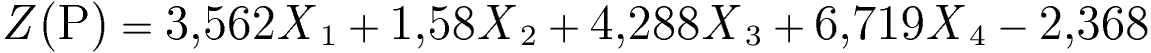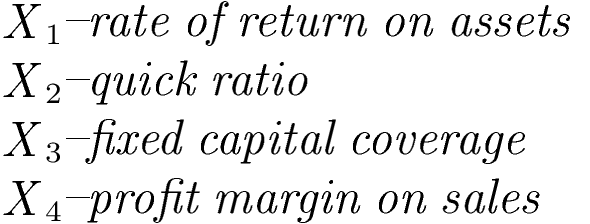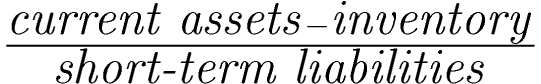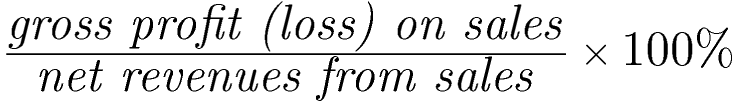Comprehensive software for monitoring and evaluation of the economic situation of the enterprise and early warning# The "Poznanski" model (2004)

Method of calculationwhere:Model description

The discriminant function of this model consists of four financial ratios that refer to profitability, financial liquidity and capital structure.

Interpretation of the Z(P)-score

• Z (P)= 0 -critical value (bankruptcy threat for the function below the critical value)

• Z (P)≤ 0 -"bankrupt" (distess zone)
• Z (P)> 0 -"non-bankrupt" (safe zone)
• Model fit: 96% (the model correctly classified 96% of the examined companies).
• The higher the Z(P) value (0.1 and more), the better is the financial situation of a company and the lower is the bankruptcy threat within the forthcoming year.
• The increase of Z(P) value over time indicates the improvement of financial situation and decrease of bankruptcy threat.
• In the case of the Z(P) < 0 (which signalizes bankruptcy threat) it is recommended to run a detailed analysis of financial performance to detect which aspects require an immediate healing procedures.

Detailed decription of respective ratios and their impact on the Z(P)-score value

Constant value -2.368

The constant, free factor results from statistical estimates when building the model, it allows setting the limit value of Z(P) at the level of zero.

X– rate of return on assets

Method of calculationRatio's weight: +3.562
Units: %

Remarks

The ratio is calculated like the return on assets (ROA) ratio available in the eanaliza.pl system in the group of profitability ratios. It uses the data for the current period.

Ratio's interpretation

• A higher value of the ratio means greater ability of the company to generate profit based on its resources (assets).
• In the model, the ratio X1 has a weight with a positive sign, which means that the increase in the ratio (increase in profitability) will increase the value of the function Z(P).
• In case of a negative result (financial loss), the ratio X1 will decrease the value of the Z(P) function, increasing the risk of bankruptcy.

X2 – quick ratio

Method of calculationRatio's weight: +1.58
Units: times

Remarks

The ratio is partially calculated like the quick ratio available in the eanaliza.pl system in the group of liquidity ratios, but in the numerator we omit the short-term prepayments. It uses the data for the current period.

Ratio's interpretation

• A higher value of the ratio means a higher level of liquidity which reduces the risk of bankruptcy.
• In the model, the Xratio has a weight with a positive sign, which means that increase in the ratio, will increase the Z(P) function, reducing the bankruptcy risk

X3 – fixed capital coverage

Method of calculationRatio's weight: +4.288
Units: times

Remarks

The ratio is partially calculated like the long term solvency ratio based on fixed capital coverage available in the eanaliza.pl system in the group of general performance ratios, but in the denominator we take the value of total assets instead of fixed assets value. It uses the data for the current period.

Ratio's interpretation

• A higher value of the ratio means higher level of long-term sources of funds (fixed capital) in financing assets, which improves firm's stability and long-term solvency.
• Higher level of fixed capital reduces the bankruptcy risk, by reducing the level of short-term liabilities (with maturity within one year).

X4 – profit margin on sales

Method of calculationRatio's weight: +6.719
Units: %

Remarks

The ratio is calculated like the gross profit margin ratio available in the eanaliza.pl system in the group of profitability ratios. It uses the data for the current period.

Ratio's interpretation

• A higher value of the ratio means greater ability of the company to generate profit based on sales.
• In the model, the ratio X4 has the highest weight with a positive sign, which means that the increase in the ratio (increase in profitability) will increase the value of the function Z(P).
• In case of a negative result (financial loss), the ratio X4 will decrease the value of the Z(P) function, increasing the risk of bankruptcy.

Based on:
HAMROL M., CZAJKA B., PIECHOCKI M. (2004). Upadłość przedsiębiorstwa-model analizy dyskryminacyjnej. Przegląd Organizacji 6, 35-39. (in Polish)
KISIELIŃSKA J., WASZKOWSKI A. (2010). Polskie modele do prognozowania bankructwa przedsiębiorstw i ich weryfikacja. Zeszyty Naukowe SGGW 82. (in Polish)

We use cookies to ensure you have the best experience on our website. If you continue to use this site, we will assume that you are happy with it.